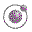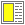Name binding, Recursion, Iteration, and Continuations - slide 14 : 42

Example with cond: as-string
```(define (as-string x)
(cond ((number? x) (number->string x))
((symbol? x) (symbol->string x))
((string? x) x)
((boolean? x)
(if x "true" "false"))   ; consider "#t" and "#f" as alternatives
((char? x) (char->string x))
((list? x)
(string-append "("
(string-merge (map as-string x) (make-list (- (length x) 1) " "))
")"))
((vector? x)
(let ((lst (vector->list x)))
(string-append "#("
(string-merge (map as-string lst) (make-list (- (length lst) 1) " "))
")")))
((pair? x)
(string-append "("
(apply string-append
(map (lambda (y) (string-append (as-string y) " ")) (proper-part x))
)
" . " (as-string (first-improper-part x))
")"))
(else "??")))```